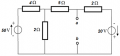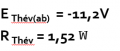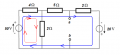# Thevenin equivalent schematic with 2 sources and junction in between

#### punctualmanx375

Joined Jun 3, 2018
11
I have to determine a Thevenin equivalent of this schematic (see attachment). (not as homework but preparation for an exam)
I've been trying for over 3 hours but have no idea how to do this.
I've tried to redraw the circuit but doesn't help much...
Any help would be appreciated.Moderators note: shown images full size

#### Jony130

Joined Feb 17, 2009
5,230
But did you manage to solve for Vth?

#### punctualmanx375

Joined Jun 3, 2018
11
Eth = Vth in the solution. I know how to draw a thevenin equivalent schematic. but what I'm asking is how did he(the teacher) get to Eth. Rth is no problem, I know how to do that but I'm stuck trying to find Vth/Eth

#### punctualmanx375

Joined Jun 3, 2018
11
not sure what you mean by the first question, i've seen kirchoff in the past but want to solve this only using thevenin.
and for the second question:
-first calculate voltage over 2ohm resitor =(50*(2/(4+2)))=16.666667V
-second calculate Vx(other 2ohm resitor) =(16.666667*(2/(5+2)))=4.7619V
so Vx=4.7619V
next?

#### Jony130

Joined Feb 17, 2009
5,230
Do you know nodal or mesh analysis? Or maybe Kirchhoff's law only?

-first calculate voltage over 2ohm resitor =(50*(2/(4+2)))=16.666667V
-second calculate Vx(other 2ohm resitor) =(16.666667*(2/(5+2)))=4.7619V
so Vx=4.7619V
next?
No, wrong.
From what I see you trying to apply a voltage divider rule. But it won't work because yor voltage divider is loaded by (5Ω + 2Ω).
And your equation apply only to unloaded voltage divider ( circuit without 5Ω + 2Ω resistor).

Try agin.

Last edited:

#### punctualmanx375

Joined Jun 3, 2018
11
owww yeah, totally missed that.
so then:
calc replacement resistor for 2ohm//7ohm = 14/9 = 1.55555556 ohm
calc voltage over replacement resitor = 50*((14/9)/((14/9)+4)) = 14V
calc voltage over 2ohm resistor = 14*(2/(2+5)) = 4V
so Vx = 4V
(i use "//" to indicate parrallel resitors)

and to answer you're first question, I think only kirchoff's law in other words current matrix and then use the current to calc all voltages.

#### Jony130

Joined Feb 17, 2009
5,230

#### punctualmanx375

Joined Jun 3, 2018
11
I thought you were going that route...then:
calc 4//2 = 1.333333 (4/3) ohm
calc voltage over 2 ohm resistor = -20*(2/(1.333333+5+2) = -4.8 ?
Vy=-4.8

#### Jony130

Joined Feb 17, 2009
5,230
Wrong. Why 2Ω in the numerator?

#### punctualmanx375

Joined Jun 3, 2018
11
I think I see what you mean,
calc 4//2 = 4/3 ohm
calc voltage over 4/3 and 5 ohm = -20*(((4/3)+5)/((4/3)+5+2)) = -15.2?
Vy = -15.2?
and if you combine 1 and 2 you get -15.2+4=-11.2?

(sorry for the long response time, only have 5 posts/hour apparently. So I had to wait...)

#### Jony130

Joined Feb 17, 2009
5,230
and if you combine 1 and 2 you get -15.2+4=-11.2?
Yep, and this the Vth voltage equal to Vth = -11.2V
And this technique of solving the circuit is called a superposition.

#### punctualmanx375

Joined Jun 3, 2018
11
oooh that rings a bell.
thanks so much!

#### Jony130

Joined Feb 17, 2009
5,230
But are you familiar with nodal or mesh analysis?

#### punctualmanx375

Joined Jun 3, 2018
11
But are you familiar with nodal or mesh analysis?
no idea, if i look it up on google mesh analysis rings a bell,
determine the currents (by formula)
then put them in a matrix and calculate?

nodal analysis I haven't heard of though

btw, any way to get rid of the 5 posts per hour?

#### Jony130

Joined Feb 17, 2009
5,230
if i look it up on google mesh analysis rings a bell,
determine the currents (by formula)
then put them in a matrix and calculate?
For such a simple circuit matrix is not neededFor blue mesh (I1) we have

(I1 + I2)*4Ω + I1*5Ω + I1*2Ω - 20V - 50V = 0

And for red mesh (I2)

(I2 + I1)*4Ω + I2*2Ω - 50V = 0

And the soution is

I1 = 4.4A
I2 = 5.4A

So Vth = I1*2Ω+(-20V) = 4.4A*2Ω-20V = -11.2V (KVL)

Last edited:

#### WBahn

Joined Mar 31, 2012
26,398
no idea, if i look it up on google mesh analysis rings a bell,
determine the currents (by formula)
then put them in a matrix and calculate?

nodal analysis I haven't heard of though

btw, any way to get rid of the 5 posts per hour?
There are too many techniques that just "ring a bell" for you to succeed. You need to stop where you are and go back and review basic circuit analysis until you are proficient using

Kirchhoff's Voltage Law
Kirchhoff's Current Law
Mesh Current Analysis
Node Voltage Analysis
Superposition

to analyze circuits containing multiple sources, including all four of the basic dependent sources.

If you don't, you will just be digging yourself a deeper and deeper hole that will be progressively more difficult to get out of.

•Jony130

#### MrAl

Joined Jun 17, 2014
8,156
Hi,

A nice way to do this is to first collapse the left side of the network (left of a,b)
into it's Thevenin equivalents and then go from there. That leaves you with two
voltage sources and just two resistors and the advantage is then we can easily
find both the Thevenin voltage and resistance just by considering those two
sources and those two resistances.

To start on the left, the Norton current is i=50/4 and that is in parallel with the
Norton resistance of 4 in parallel with 2, and 2 is like two 4 ohm resistors in parallel
so the resistance is r=4/3. i*r=50/3 so the first Thevenin voltage is v=50/3 in series
with resistance r=5+4/3=19/3.
Now to find the superposition contribution from the 50v source we just use the voltage
divider formula with upper R1=19/3 and lower R2=2 and voltage source V=50/3, and that gives
us 4 volts. To find the total Thevenin voltage we just have to consider the -20v
source next, along with the first Thevenin voltage and resistance.
Since we also have the left hand side resistance too, we can then easily find the total
Thevenin resistance by placing that in parallel with the 2 ohm resistor and that gives
us the 1.52 ohms.